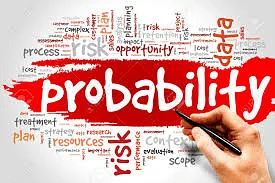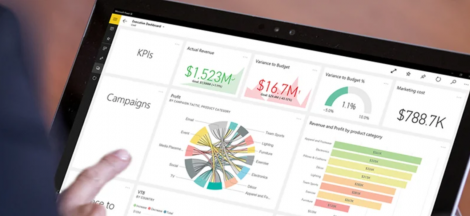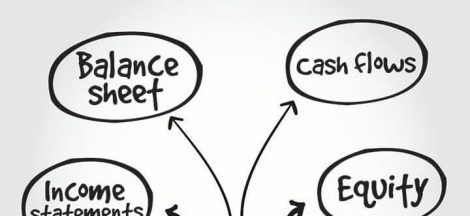# PROBABILITY FOR BEGINNERS 1.0Theory of Probability

Probability is a mathematical subject with its own set of rules, definitions, and laws for determining the likelihood of specific outcomes, occurrences, and combinations of outcomes and events.

Specifically, the study of probability is about quantitative decision-making.

The probabilities of the original outcomes and events must be added, subtracted, multiplied, or divided to determine probability. Some mixes are so common that they’ve developed their own set of rules and formulas. The better you comprehend the concepts behind the formulas, the easier it will be to recall and apply them correctly.

Probability values are always assigned on a scale from 0 to 1. Whilst a probability near zero indicates an event is quite unlikely to occur, a probability near one indicates an event is almost certain to occur.

How to Solve Probability Questions

To solve probability problems, you must first construct the problem and then subsequently deconstruct the data. The process of problem-solving is rarely simple, and mastering it takes time.

Predicting probabilities becomes a lot easier with some practice and a few pointers.

When completing a probability word problem, one vital tip to remember is to look for the keyword, which will help you figure out which rule of probability to use. “And,” “or,” and “not” are the keywords.

When dealing with problems that include the keyword “and,” a multiplication rule is the probability rule to employ. When dealing with scenarios using the term “or,” an addition rule should be applied. The complement rule is the probability rule to employ when dealing with the keyword “not.”

Probabilities are found in everyday life – from weather predictions to football outcomes; from consumer buying behaviour to probabilities of gain or loss associated with investment decisions, product design, etc. Probability theory is also applicable in the field of medical science, pharmacology, livestock breeding (Agriculture).

Assigning Probabilities

1. Classical Method: This is the traditional way of determining probability and it is used when all possible outcomes are known in advance and all outcomes are equally likely. A practical example of the classical method of probability is rolling a die.
2. Relative Frequency Method: The relative frequency technique is applied, when all possible outcomes are unknown ahead of time and are not all equally likely. This method makes use of historical data, but similar situation. A manufacturing company that increases its production capacity based on last year’s sales would utilize the relative frequency technique.
3. Subjective Method: We define probability in the subjective approach as the degree of belief we have in the occurrence of an event. As a result, attributing probability based on the subjective method relies on judgment.

In general, combining estimates from the classical or relative frequency approach with subjective judgment yields the best probability estimates.

Marginal, conditional, and joint probabilities

Marginal Probability

This is the likelihood of an event occurring regardless of the outcome of another variable. It is an unconditional probability. It is not contingent on another event happening.

Conditional Probability

This is the probability of an event occurring, given that another event has occurred. It is calculated by adopting the ‘Multiplication Law’, that is multiplying the probability of the prior event by the probability of the conditional event.

Joint Probability

It is the probability of events A and B occurring together. Joint probability is the chance that two or more events may intersect.## Cleaning your Data with Power BI

in## My understanding of Basic Corporate Financial Accounting

in